• +91 9971497814
• info@interviewmaterial.com

# RD Chapter 23- The Straight Lines Ex-23.1 Interview Questions Answers

### Related Subjects

Question 1 :
Find the slopes of the lines which make the following angles with the positive direction of x – axis:
(i) – π/4
(ii) 2π/3

(i) – π/4
Let the slope of the line be ‘m’
Where, m = tan θ
So, the slope of Line is m = tan (– π/4)
= – 1
∴ The slope of the line is – 1.
(ii) 2π/3
Let the slope of the line be ‘m’
Where, m = tan θ
So, the slope of Line is m = tan (2π/3)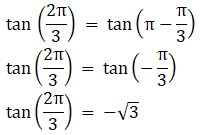∴ The slope of the line is –√3

Question 2 :

Find the slopes of a line passing through the following points :
(i) (–3, 2) and (1, 4)

(ii) (at21, 2at1) and (at22,2at2)

(i) (–3, 2) and (1, 4)

By using the formula,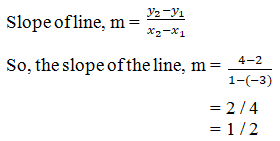The slope of the lineis ½.

(ii) (at21,2at1) and (at22, 2at2)

By using the formula,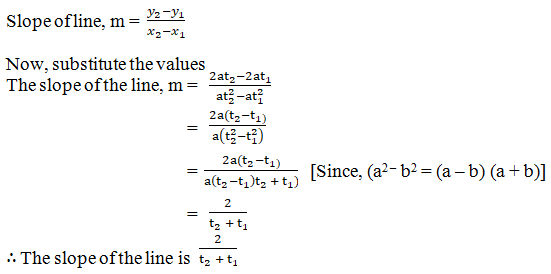Question 3 :
State whether the two lines in each of the following are parallel, perpendicular or neither:
(i) Through (5, 6) and (2, 3); through (9, –2) and (6, –5)
(ii) Through (9, 5) and (– 1, 1); through (3, –5) and (8, –3)

(i) Through (5, 6) and (2,3); through (9, –2) and (6, –5)

By using the formula,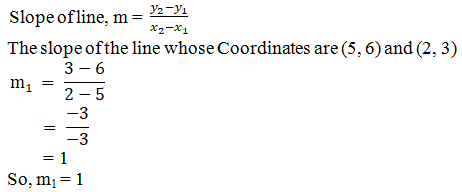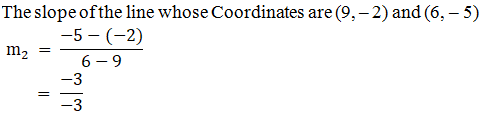So, m2 =1

Here, m1 = m=1

The lines areparallel to each other.

(ii) Through (9, 5) and (–1, 1); through (3, –5) and (8, –3)

By using the formula,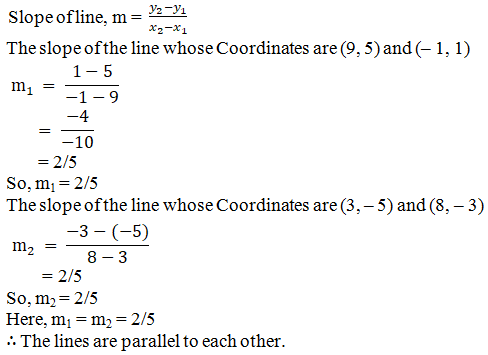Question 4 :
Find the slopes of a line
(i) which bisects the first quadrant angle
(ii) which makes an angle of 300 with the positive direction of y – axis measured anticlockwise.

(i) Which bisects thefirst quadrant angle?

We know that, if theline bisects in the first quadrant, then the angle must be between line and thepositive direction of x – axis.

Since, angle = 90/2 =45o

By using the formula,

The slope of the line,m = tan θ

The slope of the linefor a given angle is m = tan 45o

So, m = 1

The slope of the lineis 1.

(ii) Which makes anangle of 300 with the positive direction of y – axis measuredanticlockwise?

Given: The line makesan angle of 30o with the positive direction of y – axis.

We knowthat, angle between line and positive side of axis => 90o +30o = 120o

By using the formula,

The slope of the line,m = tan θ

The slope of the linefor a given angle is m = tan 120o

So, m = – √3

The slope of the lineis – √3.

Question 5 :
Using the method of slopes show that the following points are collinear:
(i) A (4, 8), B (5, 12), C (9, 28)
(ii) A(16, – 18), B(3, – 6), C(– 10, 6)

(i) A (4, 8), B (5, 12), C(9, 28)

By using the formula,

The slope of the line= [y2 – y1] / [x2 – x1]

So,

The slope of line AB =[12 – 8] / [5 – 4]

= 4 / 1

The slope of line BC =[28 – 12] / [9 – 5]

= 16 / 4

= 4

The slope of line CA =[8 – 28] / [4 – 9]

= -20 / -5

= 4

Here, AB = BC = CA

The Given points arecollinear.

(ii) A(16, – 18), B(3, –6), C(– 10, 6)

By using the formula,

The slope of the line= [y2 – y1] / [x2 – x1]

So,

The slope of line AB =[-6 – (-18)] / [3 – 16]

= 12 / -13

The slope of line BC =[6 – (-6)] / [-10 – 3]

= 12 / -13

The slope of line CA =[6 – (-18)] / [-10 – 16]

= 12 / -13

= 4

Here, AB = BC = CA

The Given points arecollinear.

Todays Deals### RD Chapter 23- The Straight Lines Ex-23.1 Contributorskrishan

Name:
Email:

# Latest News# 9000 interview questions in different categories